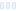# Energy Formula, Zesty Orange

## Mountain Peak Nutritionals

Main info:

Energy Formula, Zesty Orange
Mountain Peak Nutritionals
1 scoop
20.0 Calories
3.0 g
0 g
0 g

0.4 g
0 mg
0 g
90 mg
1 g
0 g

Percent calories from...
Nutrition Facts
For a Serving Size of (g)
How many calories are in Energy Formula, Zesty Orange? Amount of calories in Energy Formula, Zesty Orange: Calories Calories from Fat (%)
% Daily Value *
How much fat is in Energy Formula, Zesty Orange? Amount of fat in Energy Formula, Zesty Orange: Total Fat
How much sodium is in Energy Formula, Zesty Orange? Amount of sodium in Energy Formula, Zesty Orange: Sodium
How many carbs are in Energy Formula, Zesty Orange? Amount of carbs in Energy Formula, Zesty Orange: Carbohydrates
How many net carbs are in Energy Formula, Zesty Orange? Amount of net carbs in Energy Formula, Zesty Orange: Net carbs
How much sugar is in Energy Formula, Zesty Orange? Amount of sugar in Energy Formula, Zesty Orange: Sugar
How much fiber is in Energy Formula, Zesty Orange? Amount of fiber in Energy Formula, Zesty Orange: Fiber
How much protein is in Energy Formula, Zesty Orange? Amount of protein in Energy Formula, Zesty Orange: Protein
Vitamins and minerals
How much Vitamin A is in Energy Formula, Zesty Orange? Amount of Vitamin A in Energy Formula, Zesty Orange: Vitamin A
How much Vitamin C is in Energy Formula, Zesty Orange? Amount of Vitamin C in Energy Formula, Zesty Orange: Vitamin C
How much Calcium is in Energy Formula, Zesty Orange? Amount of Calcium in Energy Formula, Zesty Orange: Calcium
Fatty acids
Amino acids
* The Percent Daily Values are based on a 2,000 calorie diet, so your values may change depending on your calorie needs.Loading similar foods...
Note: Any items purchased after clicking our Amazon buttons will give us a little referral bonus. If you do click them, thank you!Be cool

- Zen orangeI never skip arm day

- Buff broccoli